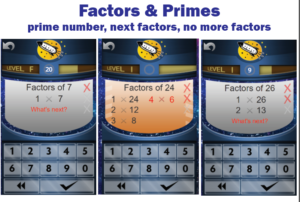## Learn three keys to knowing how to find factors and primes

We have added Factors and Primes to the Online Game. Learning Track #15 Factors & Primes is a dream come true for Dr. Don.  Many middle grades students find that factoring and finding the GCF (Greatest Common Factor) is quite difficult.  This learning track will make it easy.  It accomplishes three key learning objectives at the same time.

##(1) Recognizing the Primes.

Instead of just working on numbers with factors this Learning Track includes many prime numbers such as 7 in the illustration above.  When a number is prime, the student is shown 1 x the number and asked “What’s next?” Then the student is to answer with the check mark indicating that number only has 1 and itself as factors–there are no more. These prime numbers appear again and again, giving the student lots of practice in remembering the primes and in remembering their key characteristic.

## (2) Learning factors in order.

The game displays the factors with the lowest factor on the left and put them in order by that factor.  (see the Factors of 12 above). The factors are always in this order, so students learn them in order.  Notice that the last factors of 12 ends with 3 on the left and 4 on the right.  The factors on the right go up from there up to 12.  The key to knowing there are no more factors is when the next number down (in this case 4) is already showing in the list going up on the right.  By seeing the factors always in this order, student can more easily learn them.

## (3) Knowing when you’re done.

Sometimes we require students to indicate that all the factors have been listed. The Online Game will show all the factors and ask, “What’s next?”  Students will know that there are no more factors because they have learned them in order.  Students will then put a check mark to say this is the complete list. When figuring GCF it is essential to know when you have listed all the factors.

## 16 Learning Tracks in the Online Game

1. Addition                       5. Fact Families (+, -) to 10           9. Multiplication 10s-11s-12s
2. Subtraction                 6. Fact Families (+, -) from 11     10.  Division 10s-11s-12s
3. Multiplication             7. Add to 20, example 13+6        11.  Fact Families (x, ÷) to 20
4. Division                        8. Subtract from 20, 18-5            12 Fact Families (x, ÷) from 21      13. Identifying Fractions
14. Equivalent Fractions                   15. Primes & Factors              16. Fraction & Decimal Equivalents

## Test drive the game as a student, right NOW!

If you go to https://play.rocketmath.com          Use a login below to try any of the Learning Tracks for yourself.
 Username Pass code Account # Learning Track Test1 1212 11000 Addition (slowest speed) Test2 1212 11000 Subtraction (slow speed) Test3 1212 11000 Multiplication Test4 1212 11000 Division (fast speed) Test5 1212 11000 Fact Families (+,-) to 10 Test6 1212 11000 Fact Families (+,-) From 11 Test7 1212 11000 Add to 20 Test8 1212 11000 Subtract from 20 Test9 1212 11000 Mult 10s, 11s, 12s Test10 1212 11000 Div 10s, 11s, 12s Test11 1212 11000 Fact Families (x, ÷) to 20 Test12 1212 11000 Fact Families (x, ÷) From 21 Test13 1212 11000 Identify Fractions Test14 1212 11000 Equivalent Fractions Test15 1212 11000 Factors & Primes Test16 1212 11000 Fraction & Decimal Equivalents